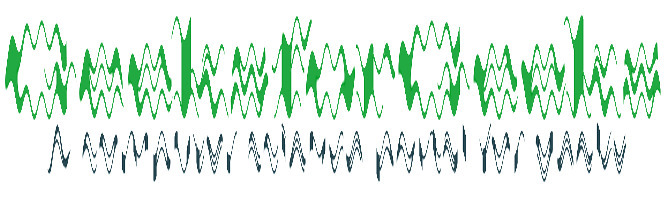Skip to content
Related Articles
Wand watermark() function – Python
• Last Updated : 10 May, 2020

The watermark() function is an inbuilt function in the Python Wand ImageMagick library which is used to transparentized the supplied image and places it over the current image, with the top left corner of the image at coordinates left, top of the current image.

Syntax:

`watermark(image, transparency, left, top)`

Parameters: This function accepts four parameters as mentioned above and defined below:

• Image: This parameter stores the image which is ti be placed.
• Transparency: This parameter stores the value of the amount of transparency. It ranges from 0.0 to 1.0.
• Left: This parameter stores the value of the x-coordinate where image will be placed.
• Top: This parameter stores the value of the the y-coordinate where image will be placed.

Return Value: This function returns the Wand ImageMagick object.

Original Image:Watermark Image:Example 1:

 `# Import library from Image ``from` `wand.image ``import` `Image`` ` `# Import the image``with Image(filename ``=``'../geeksforgeeks.png'``) as image:``    ``# Import the watermark image``    ``with Image(filename ``=``'wave1.jpg'``) as water:``        ``# Clone the image in order to process``        ``with image.clone() as watermark:``            ``# Invoke watermark function with watermark image, transparency as 0.5``            ``# left as 10 and top as 20``            ``watermark.watermark(water, ``0.5``, ``10``, ``20``)``                ``# Save the image``            ``watermark.save(filename ``=``'watermark1.jpg'``)`

Output:Example 2:

 `# Import libraries from the wand  ``from` `wand.image ``import` `Image``from` `wand.drawing ``import` `Drawing``from` `wand.color ``import` `Color`` ` `with Drawing() as draw:``    ``# Set Stroke color the circle to black``    ``draw.stroke_color ``=` `Color(``'black'``)``    ``# Set Width of the circlw to 2 ``    ``draw.stroke_width ``=` `1``    ``# Set the fill color to 'White (# FFFFFF)'``    ``draw.fill_color ``=` `Color(``'white'``)`` ` `    ``# Invoke Circle function with center at 50, 50 and radius 25``    ``draw.circle((``200``, ``200``), ``# Center point``                ``(``100``, ``100``)) ``# Perimeter point``    ``# Set the font style``    ``draw.font ``=` `'../Helvetica.ttf'``    ``# Set the font size ``    ``draw.font_size ``=` `30``     ` `    ``with Image(width ``=` `400``, height ``=` `400``, background ``=` `Color(``'# 45ff33'``)) as pic:``        ``# Set the text and its location``        ``draw.text(``int``(pic.width ``/` `3``), ``int``(pic.height ``/` `2``), ``'GeeksForGeeks !'``)``        ``# Draw the picture``        ``draw(pic)``        ``# Import the watermark image``        ``with Image(filename ``=``'wave1.jpg'``) as water:``            ``# Invoke watermark function with watermark image, transparency as 0.5``            ``# left as 10 and top as 20``            ``pic.watermark(water, ``0.5``, ``10``, ``20``)``            ``# Save the image ``            ``pic.save(filename ``=``'watermark2.jpg'``)`

Output:Attention geek! Strengthen your foundations with the Python Programming Foundation Course and learn the basics.

To begin with, your interview preparations Enhance your Data Structures concepts with the Python DS Course. And to begin with your Machine Learning Journey, join the Machine Learning – Basic Level Course

My Personal Notes arrow_drop_up